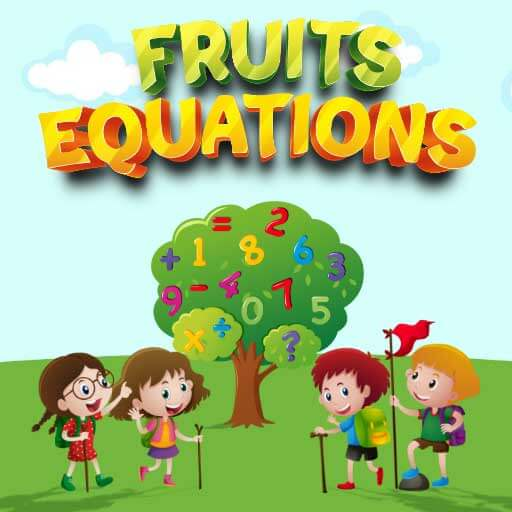## Fruits Equations## Fruits Equations

Description:

Fruits Equations is a math puzzle game. In this game you need to find the values of some fruits by solving given equations. Operations in the problems consist of addition, subtraction, multiplication and division.

Instructions:

Use mouse to select the correct answer.

Favorite:
Play it online!
Game not working?
×
Fruits Equations is not working?
* You should use least 20 words.

## Fruits Equations Video Walkthrough

Description:

Fruits Equations is a math puzzle game. In this game you need to find the values of some fruits by solving given equations. Operations in the problems consist of addition, subtraction, multiplication and division.

Instructions:

Use mouse to select the correct answer.